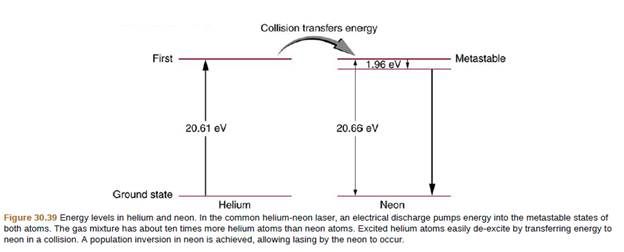Chapter 30, Problem 31PE

Chapter
Section
Textbook Problem

A helium-neon laser is pumped by electric discharge. What wavelength electromagnetic radiation would be needed to pump it? See Figure 30.39 for energy-level information.To determine

The wavelength of electromagnetic radiation that would be needed to pump when a helium-neon laser is pumped by electric discharge

Explanation

Given Data:

Given below the figure showing energy-level information. A helium-neon laser is pumped by electric discharge.

Formula Used:

Energy is given as

E=hcλ

Where, E= Energy

h= Planck's constant

c= Speed of light

λ= Wavelength

Calculation:

We have h=6.626×1034J.s , E=20.66eV

c=3×108m/sec

Substituting the values in equation of energy, we have

E=hcλ

20.66eV=(6.626× 10 34J.s)(3× 108m/sec)λ

λ=(6.626× 10 34J

Still sussing out bartleby?

Check out a sample textbook solution.

See a sample solution

The Solution to Your Study Problems

Bartleby provides explanations to thousands of textbook problems written by our experts, many with advanced degrees!

Get Started